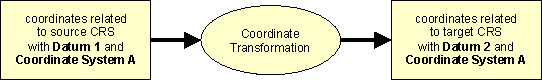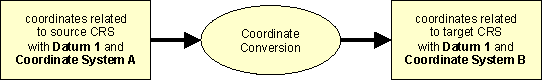﻿ CRS-EU   Changing of a CRS - Coordinate Operations Transformation and Conversion

# Changing of Coordinate Reference Systems by Coordinate Operations

## Transformation and Conversion

The changing of coordinate from one Coordinate Reference System to another is a so called coordinate operation. There exist two kinds of operations - coordinate transformation and coordinate conversion.

## Transformation

The change of coordinates from one CRS to another CRS based on different datum is only possible via a coordinate transformation. The transformation parameters could only be derived empirically by a set of points common to both coordinate reference systems it means by identical points. Choice, allocation, number and the quality of coordinates of the points affect extensive the results and the accuracy. Therefore different realisations for transformations from one datum to another exist.Schema of coordinate transformation

Generally the accuracy is for small transformation areas higher, because the strains of the two CRS are for small areas lower. But you have for each area new transformation parameters. The derivation of transformation parameters for greater areas (e.g. whole country) deliver unique results, but the accuracy is worse.

For 3-dimensional CRS in general the 7-Parameter Helmert Transformation is used for coordinate transformations. The figure shows the formula, which is used in this information system.Formula of 7 parameter Helmert transformation

Unfortunately there exists different versions of this formula with inverse definition of the signs of rotations and/or inverse sequence of the rotations. Inverse sequence means Rx = Rz, Ry = Ry, Rz = Rx. It should be considered for use of transformation parameters and software packages.

For 2-dimensional and for transformation of geo-topographical data also a grid-based transformation is usable.
A grid-based transformation based on shift values of ellipsoidal coordinates. At first in a unique previous process the shifts were determined from identical points and stored in a regular grid. The shifts for the transformation of coordinates will be computed then by bilinear interpolation inside the grid meshes.
All the complex mathematical processing was done during the creation of grid files, leaving the software to interpolate the required shifts and perform a simple addition to complete the transformation. In many cases of grid transformation the so called NTv2 - National Transformation version 2 - is in use (see NTv2 User Guide, PDF, 126kByte).

## Conversion

The change from one Coordinate System to another based on the same datum is possible via a coordinate conversion. In this case mathematical rules (e.g. map projections) are necessary. Generally this conversions are unambiguous and can realised with high accuracy.

In case of gravity related heights the conversion (e.g. normal heights to orthometric heights) requires additional information about position and gravity of the points to be transformed.Schema of coordinate conversion

## Change of CRS by Transformation and Conversion

The change of coordinates from one Coordinate Reference System to another Coordinate Reference System may result from a series of operations consisting of one or more transformations and / or one or more conversions. A concatenated operation records a change of coordinates through several transformations and / or conversions. There is no upper limit to the number of steps a concatenated operation may have. Each step is an operation described in the normal way. The figure shows as example for position the coordinate operations (conversions and transformations) for the changing of a CRS with a projected Coordinate System to another. This is in general the way for the change of a projected national CRS to an also projected pan-European CRS.Example of coordinate combined coordinate conversion and transformation